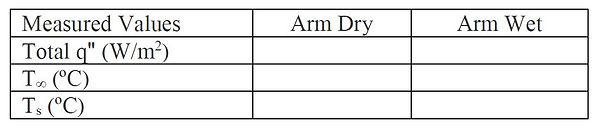###### Workshop #11 - Introduction to Water Evaporation

The human body uses water evaporation as an important heat transfer mechanism. In addition, there are many industrial processes, especially for drying of products that use evaporation processes in air.
Fortunately, convection mass transfer J can often be modeled in exactly the same way that convection heat transfer qc is modeled. In terms of the transfer per area (flux)

Heat flux is in terms of the temperature difference from the surface to the bulk fluid (air) and mass flux is in terms of the concentration difference of water vapor in air. These concentrations are equal to the density of water vapor ρ at the saturation temperature of water and can be found from steam tables. The relative humidity relates the actual water vapor concentration in air with this saturation value of water vapor all at the air temperature T

The heat transfer coefficient h and mass transfer coefficient hm can be related for the same surface by the Lewis relation

where D is the diffusion coefficient of water vapor in air and k is the thermal conductivity of air taken at the average temperature of the air

The Lewis number Le is the ratio of the thermal diffusivity of air α to the kinematic viscosity ν. For water vapor in air near room temperature conditions the Lewis number is nearly a constant value of 0.85.

This also provides an easy method to find the diffusion coefficient,

The total energy flux from the surface is the combination of the convection mass transfer and the evaporation energy flux of the mass transfer. Because the water changes from liquid to vapor during evaporation, the enthalpy of vaporization hfg must be included.

In Workshop 8 you measured convective heat transfer from your wrist. Now the challenge is to measure the combination of heat and mass transfer when sweat or water is introduced. First, tape the heat flux sensor to your wrist as before for the dry measurement. Keep the second thermocouple out in the room to record the air temperature. Take about 30 seconds of data (until steady) while moving your arm in air. Then place a wet cloth (included in your kit) that has been drenched with water over top of the sensor and repeat. The cloth dries out pretty fast so re-saturate the cloth each time before using it. Plot the heat flux as a function of time for both cases and note the change when the wet cloth is added.

Use the steady-state heat flux and temperature values to calculate the heat transfer coefficient when the arm is dry before the cloth is added. Neglect the effects of radiation. Assume the h you found stays the same (but not the surface temperature) when the cloth is added. Use this value with the Lewis Relation to calculate the corresponding mass transfer coefficient, hm. Use the temperatures of the sensor and the surrounding air to find the corresponding saturation concentrations of water vapor. Assume a relative humidity to find the water vapor concentration in the air (start with 50%). Use these values to calculate the total heat flux for the wet case. Does it match? If not, adjust the relative humidity value that you use and repeat the calculations until you get a reasonable match with your data. Record your measured values below.

Calculated value of the heat transfer coefficient for the dry case, h =

Calculate values for the wet case using the Lewis relation:

Air properties from tables at Tav:      k =                                                α =                                                 D =

Mass transfer coefficient, hm =

Properties @Ts =                               , density of water vapor ρs = Cs =                                    enthalpy of vaporization, hfg =

Guess φ =

Properties @T = . density of water vapor, ρ = C = φ ρ =

Calculated mass flux, Jʺ = hm (Cs – C) =

Calculated evaporation energy flux, Jʺ hfg = hm (Cs – C) hfg =

Calculated convection heat flux when wet, qʺc = h (Ts –T) =

Calculated wet total energy transfer, q"total = qʺc + Jʺ hfg =

Fraction of energy transfer by mass transfer =

Equations and Calculations:

###### Workshop #11 - Water Evaporation Results